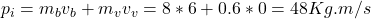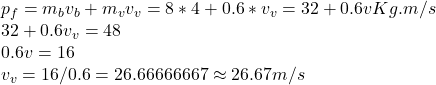## A basketball with mass of 0.8 kg is moving to the right with velocity 6 m/s and hits a volleyball with mass of 0.6 kg that stays at rest. If

Question

A basketball with mass of 0.8 kg is moving to the right with velocity 6 m/s and hits a volleyball with mass of 0.6 kg that stays at rest. If the volleyball moves to the right with velocity 4 m/s after collision, what’s the final velocity of the basketball?

in progress 0
2 weeks 2021-08-30T03:24:32+00:00 1 Answers 0 views 0

26.67 m/s

Explanation:

From the law of conservation of linear momentum, the initial sum of momentum equals the final sum.

p=mv where p is momentum, m is the mass of object and v is the speed of the object

Initial momentum

The initial momentum will be that of basketball and volleyball, Since basketball is initially at rest, its initial velocity is zeroFinal momentum